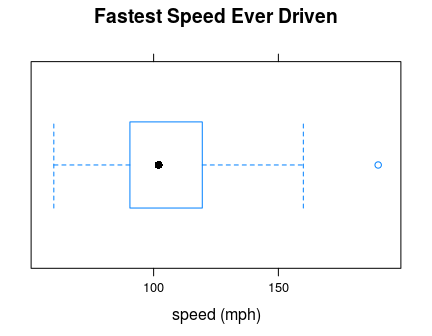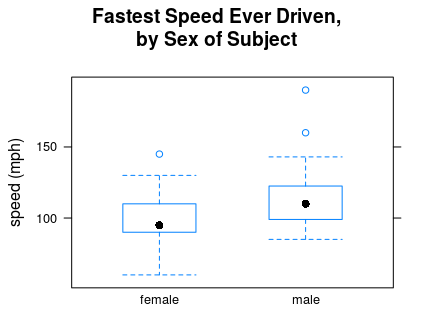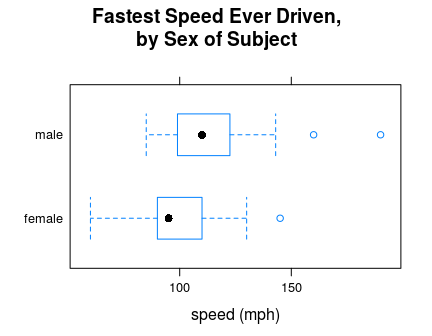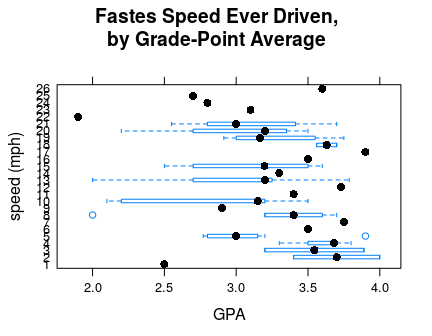# Preliminaries

The function bwplot() makes box-and-whisker plots for numerical variables. It comes from the lattice package for statistical graphics, which is pre-installed with every distribution of R. Also, package tigerstats depends on lattice, so if you load tigerstats:

require(tigerstats)

then lattice will be loaded as well.

# One Numerical Variable

For a box-and-whisker plot of the fastest speeds ever driven by students in the m111survey data frame, use the command:

bwplot(~fastest,data=m111survey,
xlab="speed (mph)",
main="Fastest Speed Ever Driven")Note the use of:

• the xlab argument to label the horizontal axis, complete with units (miles per hour);
• the main argument to provide a brief but descriptive title for the graph.

# Numerical and Factor Variable

Say you want to know:

Who tends to drive faster: the guys or the gals?

Then you are studying the relationship between the numerical variable fastest and the factor variable sex. bwplot() will break the fastest speeds up by sex and parallel box-and-whisker plots, if you run the following command:

bwplot(fastest~sex,data=m111survey,
ylab="speed (mph)",
main="Fastest Speed Ever Driven,\nby Sex of Subject")Note the use of the “” to create a two-line title. This trick can come in handy if your title is long!

If you prefer your box-and-whisker plots to be horizontal, then you can reverse the order of the variables in the formula:

bwplot(sex~fastest,data=m111survey,
xlab="speed (mph)",
main="Fastest Speed Ever Driven,\nby Sex of Subject")# Warning

Box-and-Whisker plots are great for studying:

• one numerical variable
• the relationship between a numerical variable and a factor variable.

However, if you try to study the relationship between two numerical variables with bwplot(), you will get bizarre results.

For example, suppose you want to study the relationship between fastest speed ever driven (fastest) and the grade point average (GPA) of the subjects in m111survey:

bwplot(fastest~GPA,data=m111survey,
ylab="speed (mph)",
main="Fastes Speed Ever Driven,\nby Grade-Point Average")bwplot() expects at least one of the two variable in the formula to be numerical. When it is presented with two numerical variables it politely makes do—apparently converting fastest into a new factor variable—but the resulting graph doesn’t make any sense at all.
Use xyplot() (scatterplots) to study the relationship between two numerical variables.